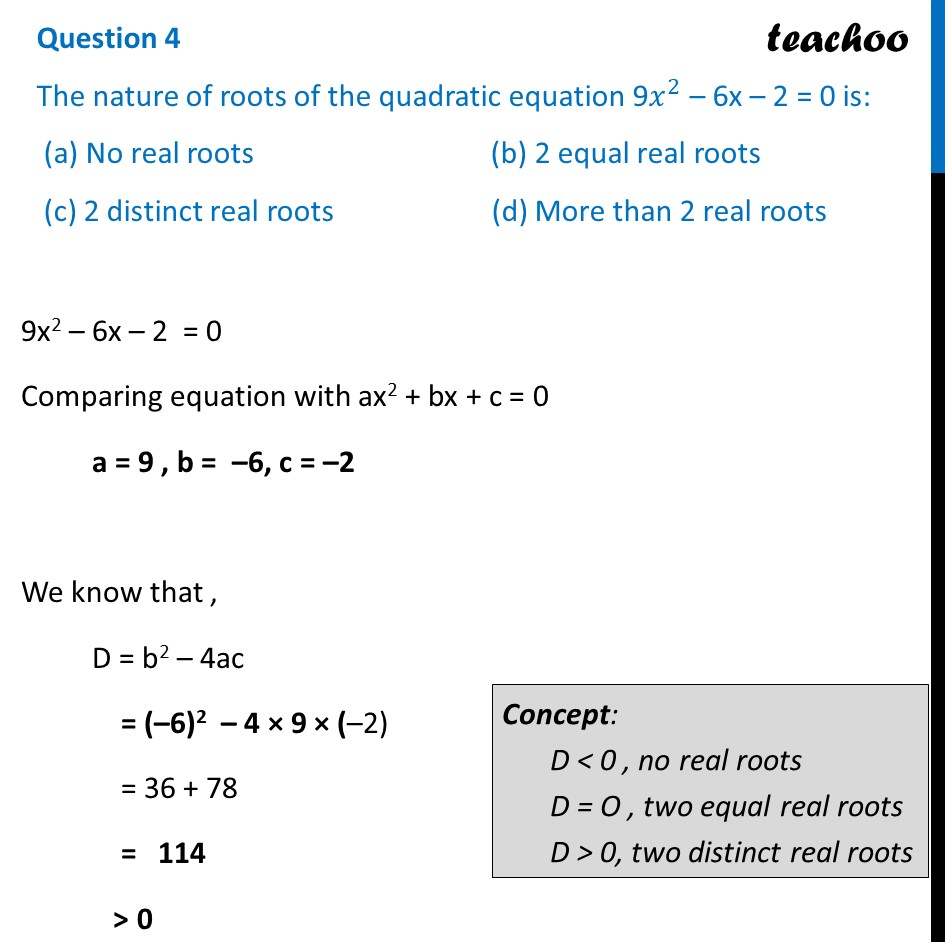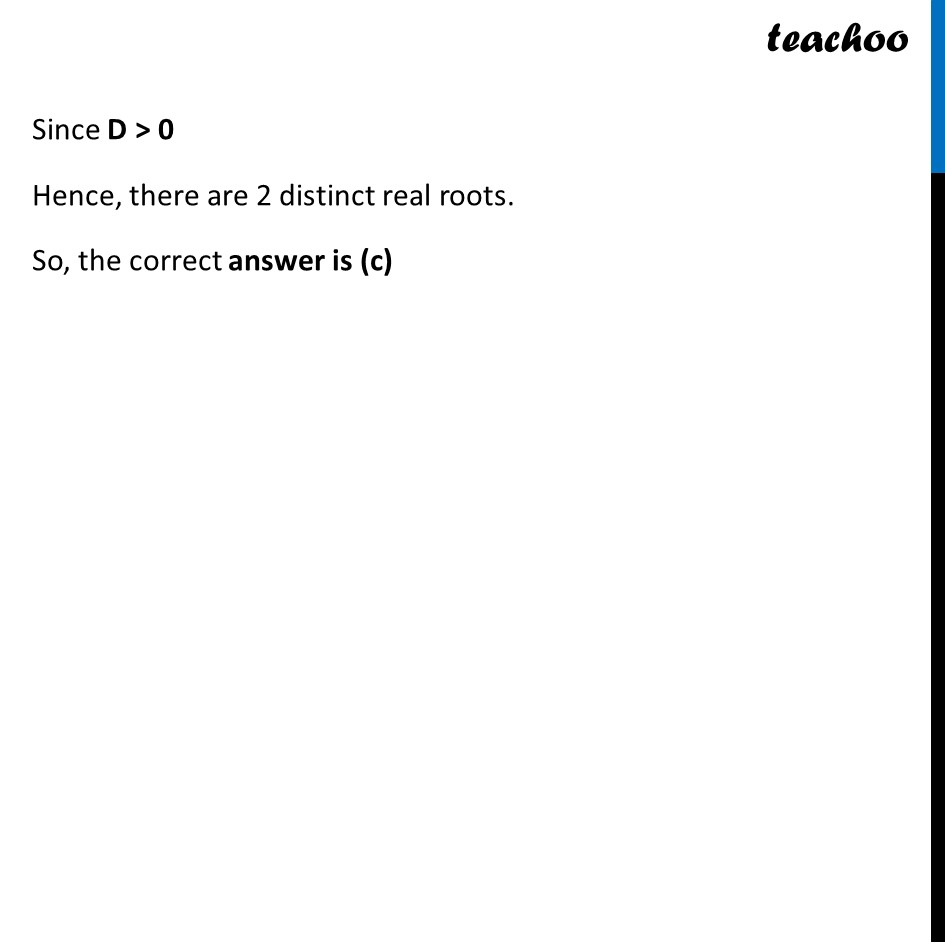CBSE Class 10 Sample Paper for 2024 Boards - Maths Standard

Class 10
Solutions of Sample Papers for Class 10 Boards

## (c) 2 distinct real roots                       (d) More than 2 real roots

This question is similar to Ex 4.3, 1 (i) Chapter 4 Class 10 Quadratic EquationsLearn in your speed, with individual attention - Teachoo Maths 1-on-1 Class

### Transcript

9x2 – 6x – 2 = 0 Comparing equation with ax2 + bx + c = 0 a = 9 , b = –6, c = –2 We know that , D = b2 – 4ac = (–6)2 – 4 × 9 × (–2) = 36 + 78 = 114 > 0 Concept: D < 0 , no real roots D = O , two equal real roots D > 0, two distinct real roots Since D > 0 Hence, there are 2 distinct real roots. So, the correct answer is (c)